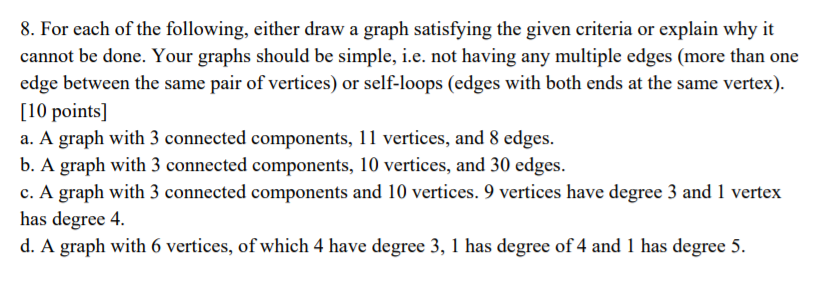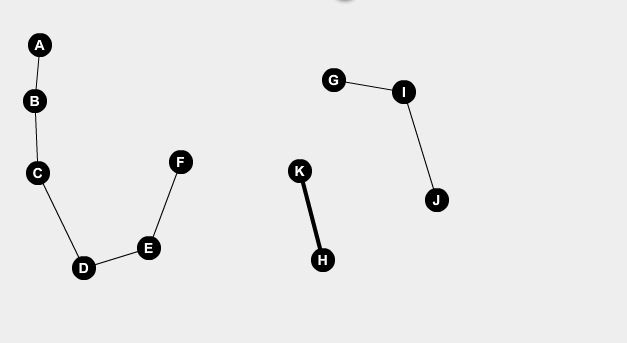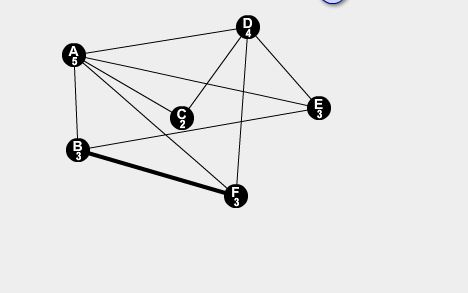# Question & Answer: For each of the following, either draw a graph satisfying the given criteria or explain why it cannot be done. Your graphs should be simple, i.e…..For each of the following, either draw a graph satisfying the given criteria or explain why it cannot be done. Your graphs should be simple, i.e. not having any multiple edges (more than one edge between the same pair of vertices) or self-loops (edges with both ends at the same vertex). a. A graph with 3 connected components, 11 vertices, and 8 edges. b. A graph with 3 connected components, 10 vertices, and 30 edges. c. A graph with 3 connected components and 10 vertices. 9 vertices have degree 3 and 1 vertex has degree 4. d. A graph with 6 vertices, of which 4 have degree 3, 1 has degree of 4 and 1 has degree 5.

Don't use plagiarized sources. Get Your Custom Essay on
Question & Answer: For each of the following, either draw a graph satisfying the given criteria or explain why it cannot be done. Your graphs should be simple, i.e…..
GET AN ESSAY WRITTEN FOR YOU FROM AS LOW AS \$13/PAGE

a-B- It is not possible as we have 10 vertices and edges need to be 30 and connected components need to be 3 we divide the vertices in pair or 6 , 3 and 1 so max edges a graph can have with n vrtices is n(n-1)/2 so with 6 vertices we have 15 edges for 3 vertices we have 3 edges and for 1 vertice we dont havee any edge so total edges comes out to be 18 . now lets try with another pair let it be 7,2,1 so for 7 we have 28 edges and for 2 we have 1 edge and for 1 we have no edge so total edges comes out to be 29 so it is not possible to draw a graph with 3 connected components 10 vertices and 30 edges,

C-it is also not possible as 9 vertices with degree 3 can be created but 1 vertex with degree 4 is not possible as we have a condition of 3 connected components so it is not possible.

D- it is also not possible the graph which is possible is 3 vertices with degree 3 1 vertex with degree 4 1 vertex with degree 5 and 1 vertex with degree 2 which is